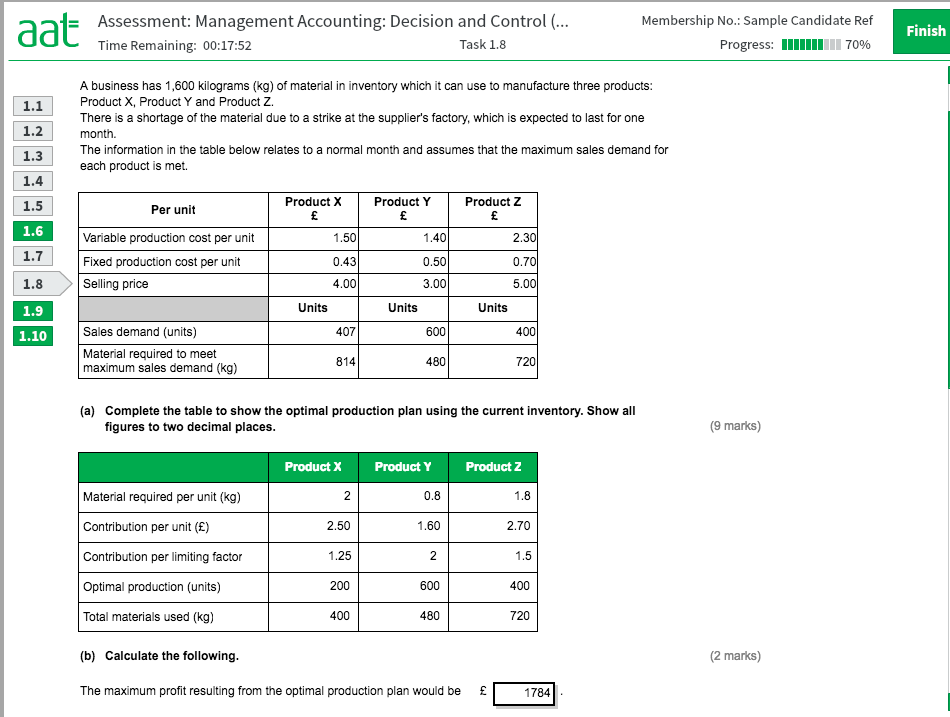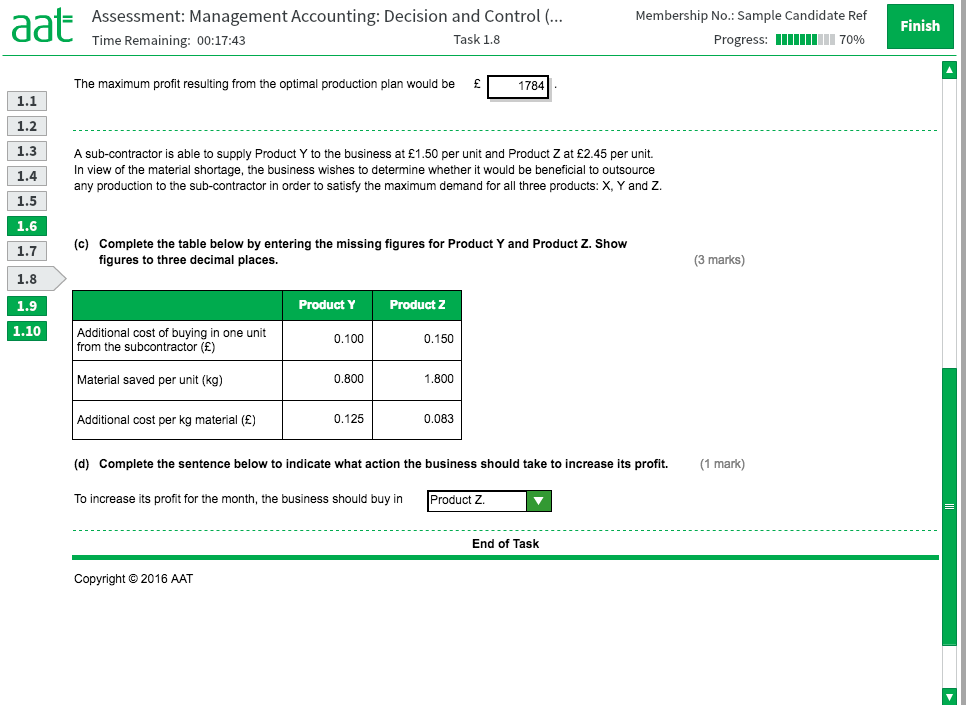# Decisions and Control - Sample Assessment 2 - Task 1.8 C and D

Hi all,

Please could someone help to explain parts C and D of the below images.

I understand the first parts A and B, but struggling with the last part.

The answers are right - I can work out where they have got the answers from, but don't fully understand why.

Apologies if it is really obvious but if someone could explain I would massively appreciate it.

Lucy• Hi Lucy,

Did you manage to find the answer to this question? I have worked out how to get the additional cost of buying in one unit, that is just the cost to supply less fixed production cost (figure shown in three decimal places) and the material saved per unit is the material required again shown in three decimal places. I am not sure where the additional cost per kg of material comes from though. Any help on this part would be appreciated.

thanks

Nikki
• Hi @Nicola_Wilson1 ,

The additional cost per kg of material is:
Y - 0.100 / 0.800 = 01.25
Z - 0.150 / 1.800 = 0.083

For Y, 0.8kg cost £0.10 For Z, 1.8kg cost £0.15 Just some maths to find the equivalent cost of 1kg

• Hi @lucym96,

In case you still need an explanations for where the figures comes from.

Additional cost of buying in. Fixed production costs will be incurred whichever the decision so ignore them.. Only consider the variable cost.

VC of Y to make is £1.40, VC to buy is £1.50, hence additional cost of buying in Y is £0.10.

To make Y 0.8kg per unit is required. Material saved per unit is the material of Y not used in production by buying in.

Additional cost per unit is as explained above. Are you okay with the division?

d) to increase profit for the month I chose the product which offered the greater contribution per unit. Y is £1.60 and Z is £2.70 so choose Z over Y.

• Thank you! I had my exam on Thursday anyway but that's a great explanation thank you!
• Thank you N4T, that makes sense :-)